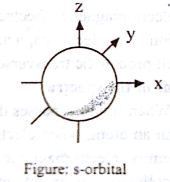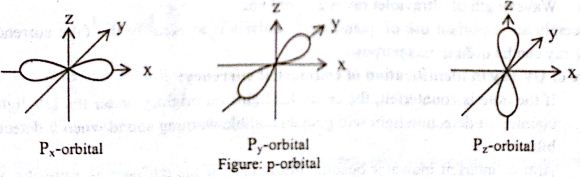# Explain on Shapes of Orbital

Shapes of orbital:

s-orbital: For s orbital, l = 0 and m = 0. This shows that the probability of locating the electron is independent of the direction from the nucleus. Consequently the shape of s orbits is spherically symmetrical. The electron cloud is not concentrated in any particular direction.p-orbital: For p orbital, since l = 1 therefore three values of m as (— 1, 0, + 1) and hence there are three p—orbital as Px, Py, Pz. All the p—orbital have same shape dumb-bell shape having two lobes of maximum probability arc directed along the three axes. All the p—orbital posses a nodel plane, i.e. a plane in which the probability of finding the electron is zero.